# Cluster Analysis of Lost Foam Casted Al-Zn-Mg-Cu Alloy with K–Mean Algorithm

Cluster Analysis of Lost Foam Casted Al-Zn-Mg-Cu Alloy with K–Mean Algorithm
Department of Mechanical Engineering, CSI Polytechnic College, Salem – 636007, Tamil Nadu, India

Department of Mechanical Engineering, Velalar College of Engineering and Technology, Erode – 638 012, Tamil Nadu, India

Department of Mechanical Engineering, K. Ramakrishnan College of Technology, Trichy – 621 112, Tamil Nadu, India

Corresponding Author Email:
vijayan.me@gmail.com
Page:
45-51
|
DOI:
https://doi.org/10.14447/jnmes.v23i1.a09
29 November 2019
|
Accepted:
17 January 2020
|
Published:
31 January 2020
| Citation
Abstract:

AA7075 is super-strength aluminum alloys to under the Al-Zn-Mg-Cu group and natural aging characteristic. The increasing need for metal matrix composites to be cost-effective and at the same time have an optimum level of performance. The contemporary market of industry-oriented software for structural strength analysis impresses very much by its versatility. The effect of conventional casting and lost foam casting of AA7075 materials mechanical properties, cluster analysis, surface roughness, and FEA analysis has been studied. The finite element analysis models were generated in ANSYS. The surface roughness of aluminum alloy 7075 made by conventional casting is lower than the by lost foam casting. From this SEM images of the aluminum alloy 7075 part made of foam casting poss's better bonding of aluminum material than conventional casting. The dense molecular bonding of aluminum in foam casting may improve the mechanical properties.

Keywords:

AA7075, foam casting, ANSYS, SEM, tensile strength.

1. Introduction

Composites are materials that exploit the combination of its constituents to raise the preferred performance characteristic. It has developed to get better the strength, stiffness, and toughness of existing materials. Based on the processing techniques are grouped into two types namely solid-state and liquid state techniques . This grouping is based on the processing temperature which is above (liquid state) or below (solid-state) the melting point of the matrix materials. The processing temperature of all processes is well below the melting point of ceramic particles. The composites are produced various traditional techniques such as stir casting, compo casting, mechanical alloying, spray deposition and powder metallurgy [2-5]. AA7075 is super strength aluminum alloys to under the Al-Zn-Mg-Cu group and natural aging characteristic. It widely used in highly stressed structures and aircraft structures application. The Al/0–30 % weight of SiC reinforced metal matrix composites fabricated by using two-step stir casting and to analyze the microstructure and dispersion of reinforced particles . The mechanical property of casted AA6061/B4C composites was evaluated. It revealed that to increasing the weight percentage of the B4C to increase the mechanical properties . The effect of alumina particle and size on the mechanical properties of the composites was modified . The wetting agent K2TiF6 is improving the interfacial bonding of the matrix materials and reinforcements . AA2014/20 vol% SiC MMC was fabricated using stir casting and examined the tool wear while machining of the composites. The industry-oriented software for structural strength analysis impresses very much by its versatility and widest functionality . The numerical modeling techniques possible of value in serving to optimize the properties and process for manufacturing improved composites. FEA was used (finite element analysis) at the micro scale and this approach proved to be quite accurate under definite situations. The micro-mechanical scratch development of composites was forecasted by various authors and the 2D axisymmetric model and 3D unit cell models to calculate the elastic nature of the composites [11-15]. 2D FEA calculations were performed to calculate quantitatively the tensile nature of composites with ceramic particles united in stripes . The orientation of the reinforcement has significant effects, both in the elastic and plastic region . The FEA models were generated in ANSYS, using scanning electron microscope images. The percentage of major failures and stress–strain responses were predicted numerically for each microstructure . It is evident from the analysis that the clustering nature of particles in the matrix dominates the failure modes of particle reinforced metal matrix composites. The objective of the present work is to analyze the different manufacturing methods are study and predict the mechanical properties of AA7075 and distinguish the cluster analysis, the failure mechanisms from SEM image based model using finite element analyses. The major failures of AA7075 properties were studied from this model.

2. Methods and Materials

The core testing materials are prepared in the foundry at Meenakshi Foundry, Madurai, India. The testing specimens are prepared from the core testing materials according to the ASTM standards. The tensile test specimen is prepared as per ASME E8 standards. The hardness values are measured in the B- scale by using a Rockwell hardness testing machine. The indenter ball diameter is 1.58 mm and the major load applied is 100 kg. The Charpy V-notch test is a standardized high strain-rate test which determines the amount of energy absorbed by a material during fracture. The Charpy V- notch test specimen is 55 x 10 x 10 mm, position of the groove from one end is 27.5 mm, depth of the groove is 2 mm and width of the groove is 4 mm. The scanning electronic microscopic (SEM) analysis is carried out to find the morphologies on the AA7075 manufactured by conventional and lost foam casting methods. The cluster analysis is used to identify the orientation of the aluminum at breaking edges in the AA7075. K-means is one of the simplest and efficient algorithms. It does solve the clustering problem using color-based segmentation. The 'MATLAB' is used for simulating the K-mean cluster algorithm. K-means clustering calculates the distances between the inputs and centres. These centres are placed in a sly way for the reason that of the different positions causes a variety of results. For the betterment, the centres have to place far away as much as possible. The new cluster centre is recalculated from Equation (1). Finally, minimizing an objective function known as the squared error function given in Equation (2).

${{\nu }_{i}}=\left( {}^{1}/{}_{{{c}_{i}}} \right)\sum\limits_{j=1}^{{{c}_{i}}}{{{x}_{i}}}$        (1)

$J(V)=\sum\limits_{i=1}^{c}{\sum\limits_{i=1}^{{{c}_{i}}}{{{\left( \left\| {{x}_{i}}-{{x}_{j}} \right\| \right)}^{2}}}}$    (2)

where, $\mathrm{c}_{\mathrm{i}}=$ number of data point in $\mathrm{i}^{\text {th }}$ cluster, $\| x_{i}$-$v_{j} \|=$ Euclidean distance between $x_{i}$ and $v_{j},$ c=Number of cluster centers.

Finite Element Analysis (FEA) software 'ABAQUS 6.14' is used for the simulation of loading and reverse loading conditions of the tensile test specimens for multiple analyses such as fully elastic material properties, elastic-plastic material properties. The test specimen is loaded in tension for elastic analysis and for elastic-plastic analysis, the tension and compression loads are considered. The mesh converging study has been carried out to determine the optimum number of elements for the analysis by varying the size of seed between 0.0012 and 0.0007. It is identified that the optimum size of the seed is 0.0008 and the number of elements is 23240. The maximum load applied to the tensile test specimen in the FEA model is 15000 N for mesh converging study. The element type of C3D8R is chosen for the analysis. It is used to determine the various parameters like Von-Mises stress, displacement, elastic and plastic strain in AA7075 materials.

## 1.pngFigure 1. Properties of aluminium alloy 7075 made by conventional casting and lost foam casting

3. Results and Discussion

Figure 1 (a) shows the average hardness values for aluminium alloy 7075 made by conventional casting and lost foam casting method. It is found that the average hardness value of aluminium alloy by lost foam casting is lower than a conventional casting. So that aluminium alloy 7075 made by the conventional casting is the harder material. Figure 1(b) shows that the surface roughness of aluminium alloy made by conventional casting is lower than the aluminium alloy 7075 by lost foam casting. Due to the presence of foam, the surface roughness is high in the lost foam casting. Figure 1 (c) shows that the impact strength for aluminium alloy 7075 made by lost foam casting is higher than the aluminium alloy made by conventional casting. Figure 1(d) shows that the tensile strength of aluminium alloy 7075 material from the lost foam casting is higher than the conventional casting.

3.1 SEM analysis

Figure 2 (a) and (b) shows the SEM image of aluminium alloy 7075 made of lost foam casting is taken at two zoom level viz 500x and 1500x respectively. In SEM image with 500x taken at a cut section of foam cast aluminum, the aluminum is distributed with close texture with 50 µm size. This is viewed in detail by the SEM image with 1500x. The image has aluminum evenly spread over the surface with a 10 µm size. The area marked in red has dense aluminum particles which strengthen the surface integrity. Figure 2 (c) and (d) show the SEM image of aluminium alloy 7075 made of conventional casting is taken at two zoom level viz 500x and 1500x respectively. In SEM image with 500x taken at a cut section of conventionally casted aluminum, the aluminum is distributed with a spider web-like texture with 50 µm size. This is viewed in detail by the SEM image with 1500x. The image has an aluminum layer with pores like over the surface with a 10 µm size. The area marked in red has aluminum particles with loose bonding which may affect the surface integrity. From this SEM images of the aluminum part made of foam casting posses better bonding of aluminum material than conventional casting. The dense molecular bonding of aluminum in foam casting may improve the impact strength. The spider web-like a form of the aluminum particle in conventional casting may yield less impact strength. The even surface texture of aluminum in foam casting may reduce its hardness and tensile strength when compared with conventional casting. The application for which the part is used may decide the demand for casting type.

3.2 Cluster Analysis Results

Cluster analysis is the task of grouping a set of substance in such a way that substances in the same group are more similar to each other than to those in other groups.

## 2.pngFigure 2. SEM image of AA 7075 - lost foam casting (a) 500x (b) 1500x and conventional casting (c) 500x (d) 1500x

## 3.pngFigure 3. SEM image of lost foam casting AA 7075 (a) Original image (1500x) and (b) Edge deduction image.

It is the main task of exploratory data mining and a common technique for statistical data analysis. The quality of a clustering technique is deliberate by its capability to find out some or all of the unseen patterns. The cluster analysis is carried out for the SEM test image of the material AA7075 manufactured in the lost foam casting and the conventional casting for the image at the high zoom level of 1500x. There are many methods of clustering developed for a wide variety of purposes. In the present study, the K-means algorithm is used to determine the natural spectral groupings present in a dataset (Image). In the K-mean algorithm, the cluster analysis is carried out for the 'K' values between 2 and 4. The simulation is carried out in the MATLAB software.

The image pattern is given as an input for the cluster analysis is shown in Figure 3(a) and the edges of the input image pattern are deducted as shown in Figure 3(b). The texture and colour feature extraction algorithm is implemented. K-means clustering algorithm is used for segmentation. Gray Level Co-occurrence Matrix (GLCM) is used for feature analysis and feature extraction.

## 4.png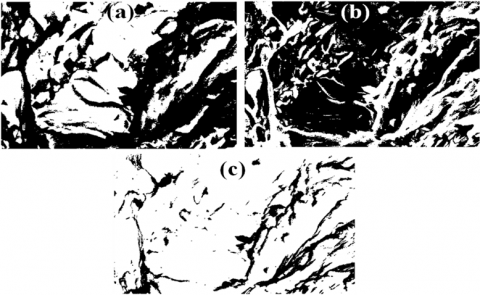Figure 4. K-means clustering image of lost foam casting at (a) K=2, (b) K=3 and (c) K=4.

## 5.png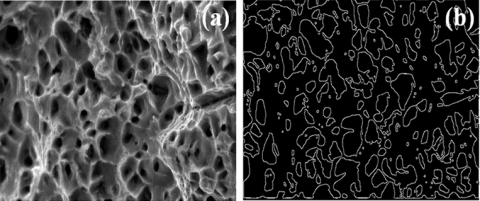Figure 5. SEM image of Conventional casting AA 707(a) Original image (1500x) and (b) Edge deduction image

## 6.pngFigure 6. K-means clustering image of conventional casting at (a) K=2, (b) K=3 and (c) K=4.

Figure 4(a), (b) and (c) show the K-means segmentation images for the different K-means such as K=2, K=3, and K=4 respectively. It is observed that for K=2 and 3 the black and white colours are equally contributed so that it's very difficult to identify the boundaries of the material texture of AA7075 manufactured in lost foam casting. For K= 4, it is identified that the white colour is more dominated than black. So that it is easy to identify the boundaries of the material texture. It revealed that the boundaries of the material texture are presented in the continuous long pattern arbitrarily in AA7075 material manufactured in the lost foam casting method. The cluster analysis is carried out for the SEM test image of the material AA7075 manufactured in the conventional casting.  It has been carried out for the image at the high zoom level of 1500x. The image pattern is given as an input for the cluster analysis is shown in Figure 5 (a) and the edges of the input image pattern are deducted as shown in Figure 5 (b). Figure 6 (a) (b) and (c) shows the K-means segmentation images for the different K-means such as K=2, K=3, and K=4 respectively. It is observed that for K=2 and 3 the black and white colours are equally contributed so that it's not easy to identify the boundaries of the material texture of AA7075 material manufactured in the conventional casting method. For K = 4, it is identified that the white colour is more dominated than black. So that it is easy to identify the boundaries of the material texture. It revealed that the boundaries of the material texture are presented as scattering form in AA7075 material manufactured in the conventional casting method.

3.2.1 Finite Element Analysis

Initially, the minimum load required for yielding of AA7075 material is estimated from the basic expressions and for this, the Von-Mises stress is calculated without considering the stress concentration factor. The calculated Von-Mises stress is compared with the simulation Von-Mises stress value. Then the stress concentration factor has been estimated, the Von-Mises stress has been calculated and determines the percentage error in the stress value. The estimated percentage error in the stress value is shown in Table 5.1. It is observed that the Von-Mises stress developed in the finite element elastic model is 0.0639 % is greater than the Von-Mises stress calculated with considering the stress concentration factor from the basic expressions. This percentage deviation is within the acceptable limit

Table 1. Comparison of Von-Mises stress - Elastic model

 FEA model Von-Mises stress (N/m2) Percentage deviation Calculated value Simulation value Elastic 165.029 x 106 1.653 x 108 0.1639 %

3.2.2 Elastic model

The elastic model simulation has been carried out for a load of 6500 N. This load is greater than the minimum load required to yield material AA7075 calculated from the basic expressions. Table 2 shows the minimum and maximum values of various parameters such as Von-Mises stress, elastic strain, plastic strain, and spatial displacement.  It is observed that the minimum and maximum plastic strain values are zero. It revealed that the model has analyzed within the elastic limit.

Table 2. Results of various parameters - Elastic model

 S. No. Parameters Minimum value Maximum value 1 Von- Mises stress (N/m2) 0 1.653 x 108 2 Elastic strain -1.271 x 10-4 2.047 x 103 3 Plastic strain 0 0 4 Spatial displacement (m) 0 1.309 x 10-4

## 7a.png## 7b.png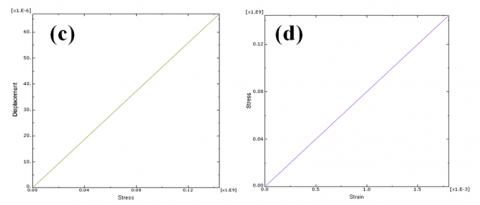Figure 7. (a) Elastic strain component (b) Spatial displacement (c) Stress Vs Displacement (d) Stress Vs Strain

Figure 7(a) shows the minimum and maximum elastic strain component of the elastic model of AA7075 material. The maximum elastic strain located at the end of reduced sections and the minimum in both end sections. It is observed that in an elastic model tensile specimen the end sections have zero strain.

Figure 7(b) shows the maximum spatial displacement at the top end section and the portion of the reduced section. The minimum displacement at the bottom end section. Figure 7(c) shows that, the relation between stress and displacement of material AA7075. It is observed that the stress is directly proportional to the displacement. This relation revealed that within the elastic limit the AA7075 material is a linear model. Figure 7(d) shows the relationship between stress and strain of material AA7075. It is identified that in material AA7075 within the elastic limit, the stress is directly proportional to the strain rate. This behavior shows that the model which has analyzed in the software 'ABAQUS' obeys Hook's law.

3.2.3 Elastic-plastic model

The elastic-plastic model simulation has been carried out for a load of 15000 N. Table 3 shows the minimum and maximum values of various parameters such as Von-Mises stress, elastic strain, plastic strain, and spatial displacement.  It is observed that in the elastic-plastic analysis the plastic strain is more than the elastic strain. It revealed that the model has analyzed within the elastic-plastic limit.

Figure 8(a) shows the minimum and maximum elastic strain component of the elastic-plastic model of AA7075 material. The maximum elastic strain located just below the end of reduced sections at the top and the minimum in both end sections. It is observed that the elastic strain is migrated toward the center of the reduced section in the specimen.  Figure 8(b) shows the plastic strain component of the specimen. It is identified that the plastic strain also migrated towards the center of the specimen. Figure 8(c) shows the spatial displacement of the specimen. The maximum displacement at the top end section and gradually it's decreased in the reduced section. The minimum displacement at the bottom end section. The displacement is zero in the bottom end section of the specimen.

Table 3. Results of various parameters (Elastic-plastic model)

 S. No. Parameters Minimum value Maximum value 1 Von- Mises stress (N/m2) 6.028 x 104 3.982 x 108 2 Elastic strain 1.125 x 10-2 -3.074 x 10-4 3 Plastic strain 0 6.219 x 10-3 4 Spatial displacement (m) 0 7.408 x 10-4

## 8.png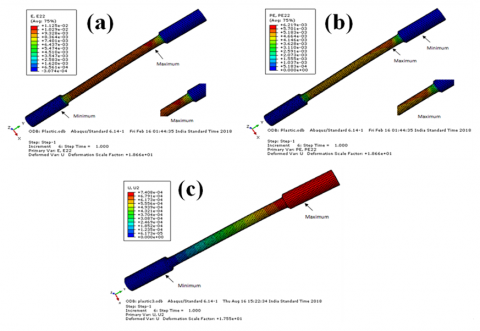Figure 8. (a) Elastic strain plot (b) Plastic strain plot (c) Spatial displacement plot

## 9.png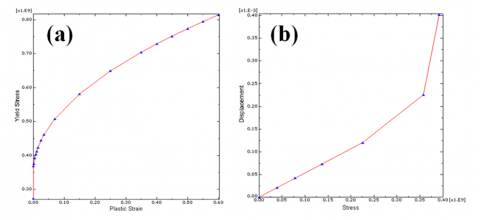Figure 9. (a) Yield stress Vs Plastic strain (b) Yield stress Vs Plastic strain

Figure 9 (a) shows the relation yield stress and plastic strain of material AA7075. It is identified that for zero plastic strain the yield stress is gradually increased and again increases by the increase in plastic strain. Figure 9 (b) shows the relationship between displacement and stress in the elastic-plastic model. It is observed that the displacement is gradually increased with an increase in the stress value. When the specimen reaches the maximum stress, the displacement is increased rapidly.

The finite element method has been used to study the reverse loading of solid homogeneous materials AA7075. The load of negative 15000 N is applied at the top side of the elastic-plastic specimen in the Reference Point (RP).  The reverse loading (compressive load) is carried out in an elastic-plastic model. In this analysis, the specimen is allowed to move in a vertically downward direction. The various parameters such as Von-Mises stress, elastic strain component, plastic strain component and spatial displacement of the material AA7075 are analyzed. The 'AC yield' is observed in the reverse loading analysis is shown in Figure 10 (a).

Table 4. Comparisons of various parameters of elastic-plastic and reverse loading models

 S. No. Parameters Reverse loading model Elastic-plastic model Percentage deviation in % Maximum value Maximum value 1 Von- Mises stress (N/m2) 3.982 x 108 3.982 x 108 0 2 Elastic strain 1.205 x 10-2 1.125 x 10-2 6.639 3 Plastic strain 6.989 x 10-3 6.219 x 10-3 11.017 4 Spatial displacement (m) 7.408 x 10-4 7.408 x 10-4 0

## 10.pngFigure 10. (a) AC YIELD plot (b) Spatial displacement Vs Simulation time period (c) Strain Vs Simulation time period (d) Stress Vs the Simulation time period

4. Conclusion

In this paper, the effect of conventional casting and lost foam casting of mechanical properties, cluster analysis, surface roughness and FEA analysis have been studied. The following conclusions have been drawn:

• The surface roughness of aluminium alloy 7075 made by conventional casting is lower than the by lost foam casting.
• The tensile strength of aluminium alloy 7075 material from the lost foam casting is higher than the conventional casting.
• From this SEM images of the aluminium alloy 7075 part made of foam casting posse’s better bonding of aluminum material than conventional casting. The dense molecular bonding of aluminum in foam casting may improve the impact strength.
• The maximum elastic strain located at the end of reduced sections and the minimum in both end sections.
References

 Sheasby, P.G., Pinner, R. (2001). The surface treatment and finishing of aluminium and its alloys, 2. ASM International.

 Williams, J.C., Starke Jr, E.A. (2003). Progress in structural materials for aerospace systems. Acta Materialia, 51(19): 5775-5799. https://doi.org/10.1016/j.actamat.2003.08.023

 Imamura, T. (1999). Current status and trend of applicable material technology for aerospace structure. Journal-Japan Institute of Light Metals, 49: 302-309.

 Fridlyander, I.N. (2000). Russian aluminum alloys for aerospace and transport applications. In Materials science forum, 331: 921-926. https://doi.org/10.4028/www.scientific.net/MSF.331-337.921

 Dinesh, S., Parameswaran, P., Vijayan, V., Thanikaikarasan, S., Rajaguru, K. (2019). Study on Microstructure and Properties of Al-Cu-Li Alloys for Electrochemical Applications Study on Microstructure and Properties of Al-Cu-Li Alloys for Electrochemical Applications, 22(1): 11 https://doi.org/10.14447/jnmes.v22i1.a03

 Saravanan, C., Dinesh, S., Sakthivel, P., Vijayan, V., Kumar, B.S. (2020). Assessment of mechanical properties of Silicon Carbide and Graphene reinforced aluminium composite. Materials Today: Proceedings, 21: 744-747. https://doi.org/10.1016/j.matpr.2019.06.751

 Xie, M., Zhu, C., Zhou, J. (2015). Mold-filling and Solidification Simulation of Grey Iron in Lost-Foam Casting. In 5th International Conference on Advanced Design and Manufacturing Engineering. Atlantis Press, 387. https://doi.org/10.2991/icadme-15.2015.78

 Fan, Z.T., Jiang, W.M., Liu, F.C., Xiao, B.T. (2014). Status quo and development trend of lost foam casting technology. China Foundry, 11(4): 296-307.

 Kownacki, A., Szarek-Gwiazda, E., Woźnicka, O. (2015). The importance of scanning electron microscopy (SEM) in taxonomy and morphology of Chironomidae (Diptera). European Journal of Environmental Sciences, 5(1): 41. https://doi.org/10.14712/23361964.2015.75

 Manoj, S., Gandhi, V.C.S. (2018). Investigation of a tool and workpiece machined using a nickel-nanocoated insert. Materiali in Tehnologije, 52(2): 171-175. https://doi.org/10.17222/mit.2017.071

 Rekha, S., Raja, V.K. (2017). Review on microstructure analysis of metals and alloys using image analysis techniques. In Frontiers in Automobile and Mechanical Engineering, IOP Conf. Series: Materials Science and Engineering, 197: 2-4. https://doi.org/10.1088/1757-899X/197/1/012010

 Mignotte, M. (2008). Segmentation by fusion of histogram-based K-means clusters in different color spaces. IEEE Transactions on Image Processing, 17(5): 780-787. https://doi.org/10.1109/TIP.2008.920761

 Gulhane, A., Paikrao, P.L., Chaudhari, D.S. (2012). A review of image data clustering techniques. International Journal of Soft Computing and Engineering, 2(1): 212-215.

 Raval, U.R., Jani, C. (2016). Implementing and Improvisation of K-means Clustering. Int. J. Comput. Sci. Mob. Comput, 5(5): 72-76.

 Yadav, A., Dhingra, S. (2016). A review on k-means clustering technique, Int. J. of Latest Research in Sci. and Tech. 5(4): 13.

 Parameswaran, P., Antony, A.G., Dinesh, S., Radhakrishnan, K. (2018). Experimental study on mechanical and corrosion characteristics of nab alloy with the addition of chromium. Materials Today: Proceedings, 5(2): 8089-8094. https://doi.org/10.1016/j.matpr.2017.11.495

 Dinesh, S., Vijayan, V., Thanikaikarasan, S., Sebastian, P.J. (2019). Productivity and Quality enhancement in Powder Mixed Electrical Discharge Machining for OHNS die steel by utilization of ANN and RSM modeling. Journal of New Materials for Electrochemical Systems, 22(1): 33-43. https://doi.org/10.14447/jnmes.v22i1.a07

 Dinesh, S., Karthikeyan, T., Vijayan, V. (2020). Powder mixed electrical discharge machining of oil hardened non shrinking steel die steel–Optimization and investigation. Materials Today: Proceedings. https://doi.org/10.1016/j.matpr.2020.04.909